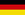Search:

» AviSynth is a powerful video FrameServer for Win32.

»Learn to script
»FAQ
»Manual
»Discussion fora
»Project page
»External filters
»FeedBack

» You can add pages to this website immediately. No login required.
Edit this document

# GeneralConvolution

( Diese Seite inDeutsch )

GeneralConvolution (clip clip, [int bias, string matrix, float divisor, bool auto])

This filter performs a matrix convolution.

 clip RGB32 clip bias (default: 0) additive bias to adjust the total output intensity matrix (default: "0 0 0 0 1 0 0 0 0") can be a 3x3 or 5x5 matrix with 9 or 25 integer numbers between 0 and 255 divisor (default: 1) divides the output of the convolution (calculated before adding bias) auto (default: true) Enables the auto scaling functionality. This divides the result by the sum of the elements of the matrix. The value of divisor is applied in addition to this auto scaling factor. If the sum of elements is zero, auto is disabled.

The divisor is usually the sum of the elements of the matrix. But when the sum is zero, you can leave divisor=1 and use the bias setting to correct the pixel values. The bias could be useful if the pixel values are negative due to the convolution. After adding a bias, the pixels are just clipped to zero (and 255 if they are larger than 255).

Some Examples:

```# Blur:
GeneralConvolution(0, "
10 10 10 10 10
10 10 10 10 10
10 10 10 16 10
10 10 10 10 10
10 10 10 10 10 ", 256, false)

# Horizontal (Sobel) edge detection:
GeneralConvolution(0, "
1  2  1
0  0  0
-1 -2 -1 ", 8)

# Vertical (Sobel) Edge Detection:
GeneralConvolution(0, "
1  0 -1
2  0 -2
1  0 -1 ", 8)

# Displacement (simply move the position of the "1" for left, right, up, down)
GeneralConvolution(0, "
0 1 0
0 0 0
0 0 0 ")

# Displacement by half pixel up (auto scaling):
GeneralConvolution(0, "
0   1   0
0   1   0
0   0   0 ")

# Displacement by half pixel to the right (manual scaling):
GeneralConvolution(0, "
0   0   0
0 128 128
0   0   0 ", 256, false)

# Sharpness filter:
GeneralConvolution(0, "
0   -1   0
-1    5  -1
0   -1   0 ", 0, true)

#In the latter example, the the new pixel values y(m,n) are given by
#y(m,n) = ( -1*x(m-1,n) - 1*x(m,n-1) + 5*x(m,n) - 1*x(m,n+1) - 1*x(m+1,n) )/1.0 + 0

# Slight blur filter with black level clipping and 25% brightening:
GeneralConvolution(-16, "
0   12   0
12  256  12
0   12   0 ", 0.75 ,true)

#In this case, the the new pixel values y(m,n) are given by
#y(m,n) = ( 12*x(m-1,n) + 12*x(m,n-1) + 256*x(m,n) + 12*x(m,n+1) + 12*x(m+1,n) )/(12+12+256+12+12)/0.75 - 16

# Emboss filter (3D relief effect)
GeneralConvolution(128, "
-1 0 0
0 0 0
0 0 1")
```

Some example can be found [here].

Changelog:

 v2.55 added divisor, auto v2 Initial ReleaseEdit this document | View document history